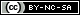# Weibull++ Examples

(Difference between revisions)
 Revision as of 23:36, 11 September 2013 (view source) (→Utilities)← Older edit Revision as of 22:09, 21 November 2013 (view source) (→Life Data Analysis)Newer edit → Line 19: Line 19: *[[Standard_Folio_Plots|Standard Folio Plots]] *[[Standard_Folio_Plots|Standard Folio Plots]] *Examples by Distribution: *Examples by Distribution: - ::*[[Weibull Distribution Examples|Weibull]] + :*[[Weibull Distribution Examples|Weibull]] - ::*[[Exponential Distribution Examples|Exponential]] + :*[[Exponential Distribution Examples|Exponential]] - ::*[[Normal Distribution Examples|Normal]] + :*[[Normal Distribution Examples|Normal]] - ::*[[Logistic_Distribution_Example|Logistic]] + :*[[Logistic_Distribution_Example|Logistic]] - ::*[[Loglogistic_Distribution_Example|Loglogistic]] + :*[[Loglogistic_Distribution_Example|Loglogistic]] - ::*[[Gamma_Distribution_Example|Gamma]] + :*[[Gamma_Distribution_Example|Gamma]] - ::*[[Generalized_Gamma_Distribution_Example|Generalized Gamma ]] + :*[[Generalized_Gamma_Distribution_Example|Generalized Gamma ]] - ::*[[Gumbel_Distribution_Example|Gumbel]] + :*[[Gumbel_Distribution_Example|Gumbel]] *[[Weibull++ Non-Parametric LDA Plot Example|Non-Parametric Life Data Analysis (LDA)]] *[[Weibull++ Non-Parametric LDA Plot Example|Non-Parametric Life Data Analysis (LDA)]] *[[Bayesian-Weibull_Lognormal_Prior Example|Bayesian-Weibull Analysis]] *[[Bayesian-Weibull_Lognormal_Prior Example|Bayesian-Weibull Analysis]] *[[Mixed Weibull Example]] *[[Mixed Weibull Example]] * Competing Failure Modes Analysis * Competing Failure Modes Analysis - ::* Two Failure Modes Example. View it in '''[http://www.reliasoft.com/Weibull/examples/rc10/index.htm HTML]''' or        '''[http://www.reliasoft.tv/weibull/appexamples/weibull_app_ex_10.html Video]''' + :* Two Failure Modes Example. View it in '''[http://www.reliasoft.com/Weibull/examples/rc10/index.htm HTML]''' or        '''[http://www.reliasoft.tv/weibull/appexamples/weibull_app_ex_10.html Video]''' - ::* [[Complex_Failure_Modes_Example|Complex Failure Modes Example]] + :* [[Complex_Failure_Modes_Example|Complex Failure Modes Example]] ==Degradation Data Analysis== ==Degradation Data Analysis==

## Quick Start Guide

The Weibull++ & ALTA Quick Start Guide has been designed to help you explore many of the software's key features by working through step-by-step instructions for some practical application examples. The guide is available as a free *.PDF download and you can also watch videos posted on ReliaSoft.tv.

## Life Data Analysis

• Crack Propagation Example (Point Estimation). View it in HTML or Video

## UtilitiesContent on this site is available/licensed under the "Creative Commons Attribution-NonCommercial-ShareAlike 4.0 International License." See Copyright Information for details.